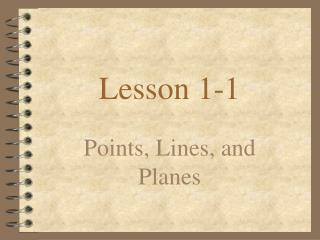DownloadDownload PresentationLesson 1-1

# Lesson 1-1

Télécharger la présentation## Lesson 1-1

- - - - - - - - - - - - - - - - - - - - - - - - - - - E N D - - - - - - - - - - - - - - - - - - - - - - - - - - -
##### Presentation Transcript

1. Lesson 1-1 Points, Lines, and Planes

2. Building Blocks of Geometry • Definition: • The three building blocks of geometry are points, lines, and planes.

3. Points Definition: It is the most basic building block of geometry. It has no size. It has only location. B A How to sketch a dot: C How to label: A * Use capital printed letters. * Never label two points with the same name (in the same sketch).

4. Lines Definition: It is straight continuous arrangement of infinitely many points. It has infinite length but no thickness. It extends forever in two directions. n NEVER ? How to sketch: ? ? • must have arrows • at both ends ? ? ? How to label: Possible Names: (Two methods) 1- small “script” letter 2- any two points on the line

5. Planes Definition: It has length and width but no thickness. It is a flat surface that extends infinitely along its length and width. horizontal “edges” M P How to sketch: N * horizontal * vertical * other vertical “edges” How to label: (Two methods) 1- capital “script” letter 2- any three points on the plane

6. Collinear Points Collinear points are points that lie on the same line. (The line does not have to be visible.) Definition: collinear non-collinear

7. Coplanar Definition: Points on the same plane Example: • Points D, E, and F are coplanar. D F E

8. Line Segment Definition: Consists of two endpoints and all points between How to sketch: How to name:

9. segments with equal lengths ( the symbol for congruent is = ) ~ Numbers are equal. Objects are congruent. AB: the distance from A to B ( a number ) AB: the segment AB ( an object ) Congruent Segments Definition: Congruent segments can be marked with . . . Correct notation: Incorrect notation:

10. Midpoint Definition: A point that divides a segment into two congruent segments.

11. Segment Bisector Definition: Any object that divides a segment into two congruent parts which is the bisector of the segment.

12. ( the symbol RA is read as “ray RA” ) Ray Definition: A ray begins at a point and extends infinitely in one direction. How to sketch: How to name: